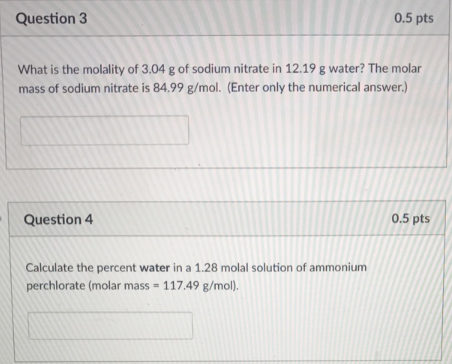# Problem: Question 3 What is the molality of 3.04 g of sodium nitrate in 12.19 g water? The molar mass of sodium nitrate is 84.99 g/mol. (Enter only the numerical answer.) Question 4 Calculate the percent water in a 1.28 molal solution of ammonium perchlorate (molar mass 117.49 g/mol).

###### FREE Expert Solution
97% (164 ratings)###### Problem Details

Question 3

What is the molality of 3.04 g of sodium nitrate in 12.19 g water? The molar mass of sodium nitrate is 84.99 g/mol. (Enter only the numerical answer.)

Question 4

Calculate the percent water in a 1.28 molal solution of ammonium perchlorate (molar mass 117.49 g/mol).